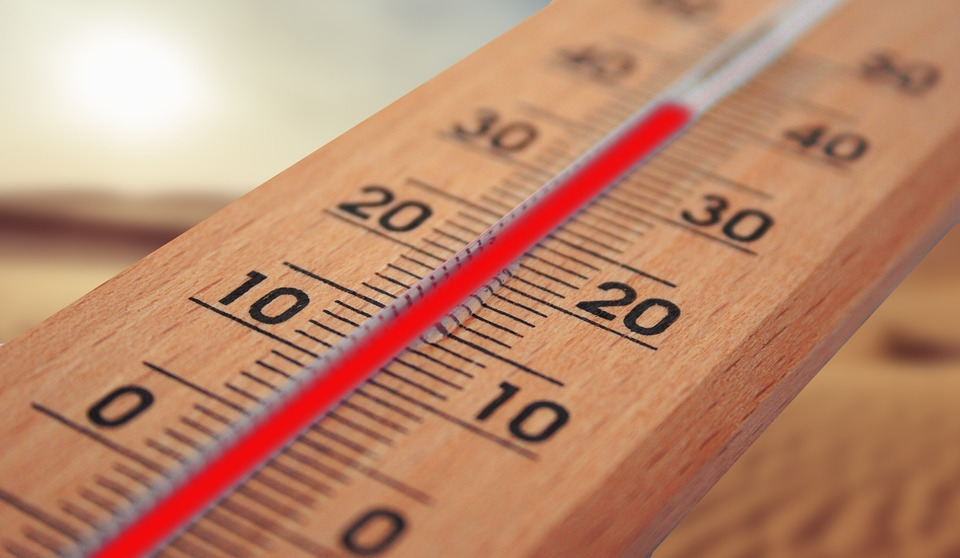# Conversion of Temperature UnitsAdvertisements
Advertisements

Related Posts:Source: https://pixabay.com/photos/thermometer-summer-heiss-heat-sun-4294021/

OpenStax Chemistry 2e

We use the word temperature to refer to the hotness or coldness of a substance. One way we measure a change in temperature is to use the fact that most substances expand when their temperature increases and contract when their temperature decreases. The mercury or alcohol in a common glass thermometer changes its volume as the temperature changes, and the position of the trapped liquid along a printed scale may be used as a measure of temperature.

Temperature scales are defined relative to selected reference temperatures: Two of the most commonly used are the freezing and boiling temperatures of water at a specified atmospheric pressure. On the Celsius scale, 0 °C is defined as the freezing temperature of water and 100 °C as the boiling temperature of water. The space between the two temperatures is divided into 100 equal intervals, which we call degrees. On the Fahrenheit scale, the freezing point of water is defined as 32 °F and the boiling temperature as 212 °F. The space between these two points on a Fahrenheit thermometer is divided into 180 equal parts (degrees).

Defining the Celsius and Fahrenheit temperature scales as described in the previous paragraph results in a slightly more complex relationship between temperature values on these two scales than for different units of measure for other properties. Most measurement units for a given property are directly proportional to one another (y = mx). Using familiar length units as one example:

where y = length in feet, x = length in inches, and the proportionality constant, m, is the conversion factor. The Celsius and Fahrenheit temperature scales, however, do not share a common zero point, and so the relationship between these two scales is a linear one rather than a proportional one (y = mx + b). Consequently, converting a temperature from one of these scales into the other requires more than simple multiplication by a conversion factor, m, it also must take into account differences in the scales’ zero points (b).

The linear equation relating Celsius and Fahrenheit temperatures is easily derived from the two temperatures used to define each scale. Representing the Celsius temperature as x and the Fahrenheit temperature as y, the slope, m, is computed to be:

The y-intercept of the equation, b, is then calculated using either of the equivalent temperature pairs, (100 °C, 212 °F) or (0 °C, 32 °F), as:

The equation relating the temperature (T) scales is then:

An abbreviated form of this equation that omits the measurement units is:

Rearrangement of this equation yields the form useful for converting from Fahrenheit to Celsius:

The SI unit of temperature is the kelvin (K). Unlike the Celsius and Fahrenheit scales, the kelvin scale is an absolute temperature scale in which 0 (zero) K corresponds to the lowest temperature that can theoretically be achieved. Since the kelvin temperature scale is absolute, a degree symbol is not included in the unit abbreviation, K. The early 19th-century discovery of the relationship between a gas’s volume and temperature suggested that the volume of a gas would be zero at −273.15 °C. In 1848, British physicist William Thompson, who later adopted the title of Lord Kelvin, proposed an absolute temperature scale based on this concept (further treatment of this topic is provided in this text’s chapter on gases).

The freezing temperature of water on this scale is 273.15 K and its boiling temperature is 373.15 K. Notice the numerical difference in these two reference temperatures is 100, the same as for the Celsius scale, and so the linear relation between these two temperature scales will exhibit a slope of

Following the same approach, the equations for converting between the kelvin and Celsius temperature scales are derived to be:

The 273.15 in these equations has been determined experimentally, so it is not exact. The image below shows the relationship among the three temperature scales.The Fahrenheit, Celsius, and kelvin temperature scales are compared. Source: OpenStax Chemistry 2e

Although the kelvin (absolute) temperature scale is the official SI temperature scale, Celsius is commonly used in many scientific contexts and is the scale of choice for nonscience contexts in almost all areas of the world. Very few countries (the U.S. and its territories, the Bahamas, Belize, Cayman Islands, and Palau) still use Fahrenheit for weather, medicine, and cooking.

EXAMPLE 1 Conversion from Celsius

Normal body temperature has been commonly accepted as 37.0 °C (although it varies depending on time of day and method of measurement, as well as among individuals). What is this temperature on the kelvin scale and on the Fahrenheit scale?

Solution

EXAMPLE 2 Conversion from Fahrenheit

Baking a ready-made pizza calls for an oven temperature of 450 °F. If you are in Europe, and your oven thermometer uses the Celsius scale, what is the setting? What is the kelvin temperature?

Solution

Source:

Source:

Flowers, P., Theopold, K., Langley, R., & Robinson, W. R. (2019, February 14). Chemistry 2e. Houston, Texas: OpenStax. Access for free at: https://openstax.org/books/chemistry-2e

Advertisements
Advertisements
Advertisements
Advertisements